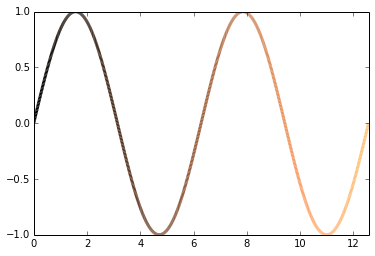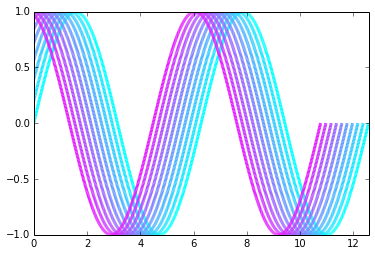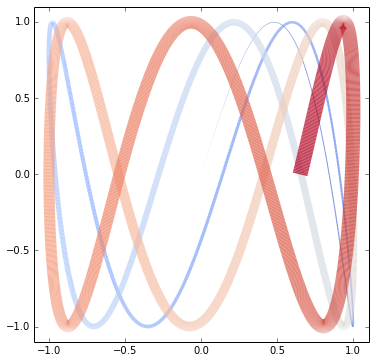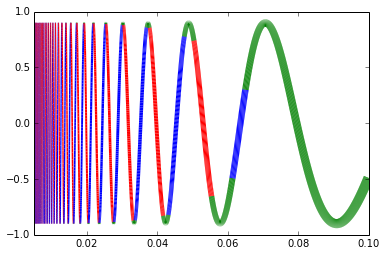# Examples of plotting (multi-)colored lines¶

Plotting multi-colored lines may be achieved using LineCollection. These examples use a more simple interface by introducing a function colorline.

In :
%pylab inline
# %config InlineBackend.figure_format = 'retina'  # Use for retina display resolution

Welcome to pylab, a matplotlib-based Python environment [backend: module://IPython.kernel.zmq.pylab.backend_inline].

In :
# Different styling for graphs (requires separate mpltools package)
# from mpltools import style
# style.use("ggplot")


## Function definitions¶

In :
# Topics: line, color, LineCollection, cmap, colorline, codex
'''
Defines a function colorline that draws a (multi-)colored 2D line with coordinates x and y.
The color is taken from optional data in z, and creates a LineCollection.

z can be:
- empty, in which case a default coloring will be used based on the position along the input arrays
- a single number, for a uniform color [this can also be accomplished with the usual plt.plot]
- an array of the length of at least the same length as x, to color according to this data
- an array of a smaller length, in which case the colors are repeated along the curve

The function colorline returns the LineCollection created, which can be modified afterwards.

'''

import numpy as np
import matplotlib.pyplot as plt
from matplotlib.collections import LineCollection
from matplotlib.colors import ListedColormap, BoundaryNorm

# Data manipulation:

def make_segments(x, y):
'''
Create list of line segments from x and y coordinates, in the correct format for LineCollection:
an array of the form   numlines x (points per line) x 2 (x and y) array
'''

points = np.array([x, y]).T.reshape(-1, 1, 2)
segments = np.concatenate([points[:-1], points[1:]], axis=1)

return segments

# Interface to LineCollection:

def colorline(x, y, z=None, cmap=plt.get_cmap('copper'), norm=plt.Normalize(0.0, 1.0), linewidth=3, alpha=1.0):
'''
Plot a colored line with coordinates x and y
Optionally specify colors in the array z
Optionally specify a colormap, a norm function and a line width
'''

# Default colors equally spaced on [0,1]:
if z is None:
z = np.linspace(0.0, 1.0, len(x))

# Special case if a single number:
if not hasattr(z, "__iter__"):  # to check for numerical input -- this is a hack
z = np.array([z])

z = np.asarray(z)

segments = make_segments(x, y)
lc = LineCollection(segments, array=z, cmap=cmap, norm=norm, linewidth=linewidth, alpha=alpha)

ax = plt.gca()

return lc

def clear_frame(ax=None):
# Taken from a post by Tony S Yu
if ax is None:
ax = plt.gca()
ax.xaxis.set_visible(False)
ax.yaxis.set_visible(False)
for spine in ax.spines.itervalues():
spine.set_visible(False)


## Example 1: Sine wave colored by time (uses the defaults for colorline)¶

In :
# Sine wave colored by time

x = np.linspace(0, 4.*np.pi, 1000)
y = np.sin(x)

fig, axes = plt.subplots()

colorline(x, y)

plt.xlim(x.min(), x.max())
plt.ylim(-1.0, 1.0)
plt.show()## Example 2: Different colors for shifted sine waves¶

In :
# Shifting a sine wave: Example modified from mpltools by Tony S Yu

x = np.linspace(0, 4.*np.pi, 1000)
y = np.sin(x)

fig, axes = plt.subplots()

N = 10
for i in xrange(N):
color = i / float(N)
shift = 0.2 * i
colorline(x-shift, y, color, cmap="cool")

plt.xlim(x.min(), x.max())
plt.ylim(-1.0, 1.0)
plt.show()## Example 3: A polar function, plotted with a custom colored dash style¶

In :
# Polar function:

max_t = 6*np.pi
theta = np.linspace(0, max_t, 1000)

alpha = 0.3
r = 1 + alpha*np.sin(7./3. * theta)

x = r * np.sin(theta)
y = r * np.cos(theta)

colored_dashes = [0.1]*8 + [0.5]*4 + [0.8]*2  # uses Python list syntax

fig, axes = plt.subplots(figsize=(6,6))

colorline(x, y, colored_dashes, cmap='jet', linewidth=8) #, [0.3]*8+[0.4]*4+[0.5]*2)

plt.xlim(x.min() - 0.1, x.max() + 0.1)
plt.ylim(y.min() - 0.1, y.max() + 0.1)

plt.axis('equal')
clear_frame()
plt.show()## Example 4: A non-periodic Lissajous curve, colored with a continuous palette and different line widths¶

In :
# Non-periodic Lissajous curve

max_t = 15.
t = np.linspace(0, max_t, 5000)
x = np.sin(t)
y = np.sin(t * np.pi)

fig, axes = plt.subplots(figsize=(6,6))

lc = colorline(x, y, alpha=0.75, linewidth=t, cmap='coolwarm')
# We can capture the LineCollection created to modify it later, e.g.:
# lc.set_rasterized = False

plt.xlim(x.min() - 0.1, x.max() + 0.1)
plt.ylim(y.min() - 0.1, y.max() + 0.1)

# plt.colorbar()  # Does not work at the moment!
plt.show()## Example 5: $\sin(1/x)$, colored according to its derivative and plotted using logarithmic spacing¶

In :
# sin(1/x), colored according to the value of its derivative

# Use logarithmic spacing so that looks better:
log_x = np.linspace(np.log(0.005), np.log(0.1), 5000)
x = np.exp(log_x)

y = 0.9 * np.sin(1. / x)

# Use finite differences to calculate the derivative of y:
y_deriv = (y[1:] - y[:-1]) / (x[1:] - x[:-1])

# Create a colormap for red, green and blue:
cmap = ListedColormap(['r', 'g', 'b'])

# Create a 'norm' (normalizing function) which maps data values to the interval [0,1]:
norm = BoundaryNorm([-1000, -200, 200, 1000], cmap.N)  # cmap.N is number of items in the colormap

fig, axes = plt.subplots()

colorline(x, y, y_deriv, cmap=cmap, norm=norm, linewidth=100.*x)
# This works even though y_deriv is a different length -- wraps around

plt.xlim(x.min(), x.max())
plt.ylim(-1.0, 1.0)
plt.show()